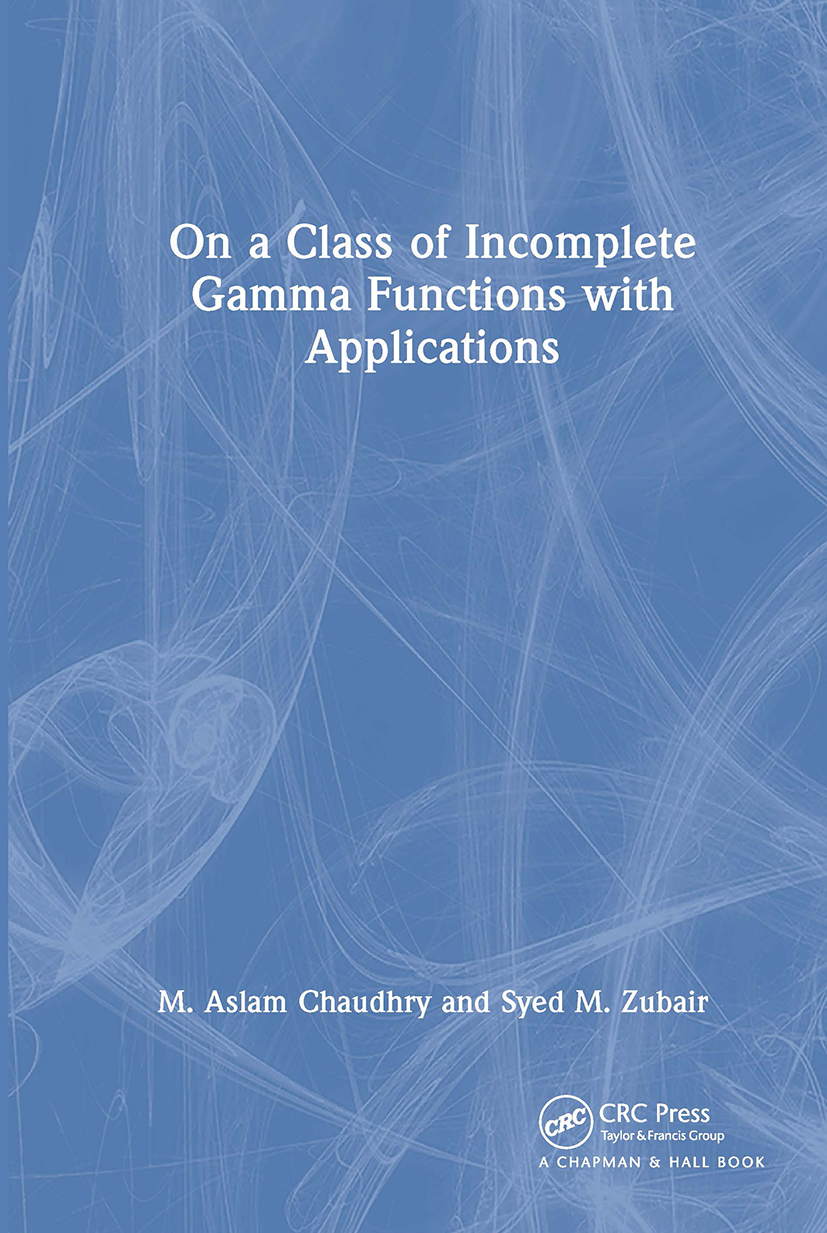# On a Class of Incomplete Gamma Functions with Applications

## 1st Edition

Chapman and Hall/CRC

512 pages

Hardback: 9781584881438
pub: 2001-08-21
\$175.00
x
eBook (VitalSource) : 9780429123627
pub: 2001-08-21
from \$28.98

FREE Standard Shipping!

### Description

The subject of special functions is rich and expanding continuously with the emergence of new problems encountered in engineering and applied science applications. The development of computational techniques and the rapid growth in computing power have increased the importance of the special functions and their formulae for analytic representations. However, problems remain, particularly in heat conduction, astrophysics, and probability theory, whose solutions seem to defy even the most general classes of special functions.

On a Class of Incomplete Gamma Functions with Applications introduces a class of special functions, developed by the authors, useful in the analytic study of several heat conduction problems. It presents some basic properties of these functions, including their recurrence relations, special cases, asymptotic representations, and integral transform relationships. The authors explore applications of these generalized functions to problems in transient heat conduction, special cases of laser sources, and problems associated with heat transfer in human tissues. They also discuss applications to astrophysics, probability theory, and other problems in theory of functions and present a fundamental solution to time-dependent laser sources with convective-type boundary conditions.

Appendices include an introduction to heat conduction, Fourier conduction, a table of Laplace transforms, and well-known results regarding the improper integrals. Filled with tabular and graphical representations for applications, this monograph offers a unique opportunity to add to your mathematical toolbox a new and useful class of special functions.

GENERALIZED GAMMA FUNCTION

The Gamma Fun ction G(a)

Definition of the Generalized Gamma Function

Properties of the Generalized Gamma Function

Mellin and Laplace Transforms

Asymptotic Representations

The Macdonald Function

The Digamma Function y(x)

Generalization of Psi (Digamma) Function

Integral Representations of yb (a)

Properties of the Generalized Psi Function

Graphical and Tabular Representations

THE GENERALIZED INCOMPLETE GAMMA FUNCTIONS

The Incomplete Gamma Functions

Definition of the Generalized Incomplete Gamma Functions

Properties of the Incomplete Generalized Gamma Functions

Convolution Representations and Laplace Transforms

Connection with Other Special Functions

KdF Functions and Incomplete Integrals

Representation in terms of KdF Functions

Reduction Formulas for F0:2;1 2:0;0[x,y]

Integrals of Product of Bessel and Gamma Functions

Asymptotic Representations

Integral Representation for G(a,x;b)

Graphical and Tabular Representations

THE FAMILY OF THE GAMMA FUNCTIONS

The Family of Incomplete Gamma Functions

The Generalized Error Functions

The Generalized Exponential Integral function

The Generalized Fresnel Integrals

The Decomposition Functions

The Extended Decomposition functions

The E(u,v) and F(u,v) Functions

The e(u) and f(u) Functions

Graphical and Tabular Representations

EXTENSION OF GENERALIZED INCOMPLETE GAMMA FUNCTIONS

Introduction

The Decomposition Formula

Recurrence Relations

Laplace and K-Transform Representation

Parametric Differentiation and Integration

Connection with Other Special Functions

Integral Representations

Differential Representations

The Mellin Transform Representation

EXTENDED BETA FUNCTION

The Beta Function

The Incomplete Beta Function

The Beta Probability Distribution

Definition of the Extended Beta Function

Properties of the Extended Beta Function

Integral Representations of the Extended Beta Function

Conncection with Other Special Functions

Representations in Terms of Whittaker functions

Extended Incomplete Beta Function

The Extended Beta Distribution

Graphical and Tabular Representations

EXTENDED INCOMPLETE GAMMA FUNCTIONS

Introduction

Definition of the Extended Incomplete Gamma Functions

The Decomposition Formula

Recurrence Formula

Connection with Other Special Functions

The H-Function

Incomplete Fox H-Functions

EXTENDED RIEMANN ZETA FUNCTIONS

Introduction

Bernoulli's Numbers and Polynomials

The Zeta Function

Zeros of the Zeta Function and the Function p(x)

The Extended Zeta Function z(a)

The Second Extended Zeta Function zb*(a)

The Hurwitz Zeta Function

Extended Hurwitz Zeta Functions

Extended Hurwitz Formulae

Graphical and Tabular Representations

PHASE-CHANGE HEAT-TRANSFER

Introduction

Constant Temperature Boundary Conditions

Convective Boundary Conditions

Freezing of Tissues around a Capillary Tube

Freezing of Binary Alloys

Freezing Around an Impurity

Numerical Methods for Phase-Change Problems

HEAT CONDUCTION WITH TIME-DEPENDENT BOUNDARY CONDITIONS

Introduction

Time-Dependent Surface Temperatures

Time-Dependent Surface Heat Fluxes

Illustrative Example

HEAT CONDUCTION DUE TO TIME-DEPENDENT LASER SOURCES

Introduction

Mathematical Formulation

Some Cases of Practical Interest

A UNIFIED APPROACH TO HEAT SOURCE PROBLEMS

Introduction

Thermal Explosions

Continuously Operating Heat Sources

APPENDICES

Heat Conduction

Table of Laplace Transforms

Integrals Dependent of Parameters

REFERENCES

SYMBOLS

INDEX